### Example 25.1 Surface Fitting with Many Noisy Variables

This example shows how you can use PROC ADAPTIVEREG to fit a surface model from a data set that contains many nuisance variables.

Consider a simulated data set that contains a response variable and 10 continuous predictors. Each continuous predictor is sampled independently from the uniform distribution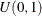. The true model is formed by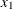and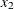: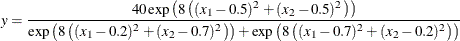The values of the response variable are generated by adding errors from the standard normal distribution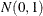to the true model. The generating mechanism is adapted from Gu et al. (1990). There are 400 generated observations in all. The following statements create an artificial data set:

data artificial;
drop i;
array X{10};
do i=1 to 400;
do j=1 to 10;
X{j} = ranuni(1);
end;
Y = 40*exp(8*((X1-0.5)**2+(X2-0.5)**2))/
(exp(8*((X1-0.2)**2+(X2-0.7)**2))+
exp(8*((X1-0.7)**2+(X2-0.2)**2)))+rannor(1);
output;
end;
run;


The standard deviation for the response without noise is 3, whereas the standard deviation for the error term is 1. So the response variable Y has a signal-to-noise ratio of 3. When eight more variables are introduced, it is harder to search for the true model because of the extra variability that the nuisance variables create. The objective is to fit a nonparametric surface model that can well approximate the true model without experiencing much interference from the nuisance variables.

The following statements invoke the ADAPTIVEREG procedure to fit the model:

ods graphics on;

model y=x1-x10;
run;


The PLOTS= FIT option in the PROC ADAPTIVEREG statement requests a fit plot. PROC ADAPTIVEREG might not produce the fit plot because the number of predictors in the final model is unknown. If the final model has no more than two variables, then the fit can be graphically presented.

PROC ADAPTIVEREG selects the two variables that form the true model (X1, X2) and does not include other nuisance variables. The "Fit Statistics" table (Output 25.1.1) lists summary statistics of the fitted surface model. The model has 27 effective degrees of freedom and 14 basis functions formed by X1 or X2 or both. The fit statistics suggest that this is a reasonable fit.

Output 25.1.1: Fit Statistics

Fit Statistics
GCV 1.55656
GCV R-Square 0.86166
Effective Degrees of Freedom 27
R-Square 0.87910
Mean Square Error 1.40260
Average Square Error 1.35351

Output 25.1.2 lists both parameter estimates and construction components (parent basis function, new variable, and optimal knot for the new variable) for the basis functions.

Output 25.1.2: Parameter Estimates

Regression Spline Model after Backward Selection
Name Coefficient Parent Variable Knot
Basis0 12.3031   Intercept
Basis1 13.1804 Basis0 X1 0.05982
Basis3 -23.4892 Basis0 X2 0.1387
Basis4 -171.03 Basis0 X2 0.1387
Basis5 -86.1867 Basis3 X1 0.6333
Basis7 -436.86 Basis4 X1 0.5488
Basis8 397.18 Basis4 X1 0.5488
Basis9 11.4682 Basis1 X2 0.6755
Basis10 -19.1796 Basis1 X2 0.6755
Basis13 126.84 Basis11 X1 0.6018
Basis14 40.8134 Basis11 X1 0.6018
Basis15 22.2884 Basis0 X1 0.7170
Basis17 -53.8746 Basis12 X1 0.2269
Basis19 598.89 Basis4 X1 0.2558

Output 25.1.3 shows all the ANOVA functional components that form the final model. The function estimate consists of two basis functions for each of X1 and X2 and nine bivariate functions of both variables. Because the true model contains the interaction between X1 and X2, PROC ADAPTIVEREG automatically selects many interaction terms.

Output 25.1.3: ANOVA Decomposition

ANOVA Decomposition
Functional
Component
Number of
Bases
DF Change If Omitted
Lack of Fit GCV
X1 2 4 405.18 1.1075
X2 2 4 947.87 2.6348
X2 X1 9 18 2583.21 6.6187

To compute predictions for the contour plot of the fitted model, you can use the SCORE statement. The following statements produce the graph that shows both the true model and the fitted model:

data score;
do X1=0 to 1 by 0.01;
do X2=0 to 1 by 0.01;
Y=40*exp(8*((X1-0.5)**2+(X2-0.5)**2))/
(exp(8*((X1-0.2)**2+(X2-0.7)**2))+
exp(8*((X1-0.7)**2+(X2-0.2)**2)));
output;
end;
end;
run;

proc adaptivereg data=artificial;
model y=x1-x10;
score data=score out=scoreout;
run;

%let off0 = offsetmin=0 offsetmax=0;
%let off0 = xaxisopts=(&off0) yaxisopts=(&off0);
%let eopt = location=outside valign=top textattrs=graphlabeltext;
proc template;
define statgraph surfaces;
begingraph / designheight=360px;
layout lattice/columns=2;
layout overlay / &off0;
entry "True Model" / &eopt;
contourplotparm z=y y=x2 x=x1;
endlayout;
layout overlay / &off0;
entry "Fitted Model" / &eopt;
contourplotparm z=pred y=x2 x=x1;
endlayout;
endlayout;
endgraph;
end;
run;

proc sgrender data=scoreout template=surfaces;
run;


Output 25.1.4 displays surfaces for both the true model and the fitted model. The fitted model approximates the underlying true model well.

Output 25.1.4: True Model and Fitted Model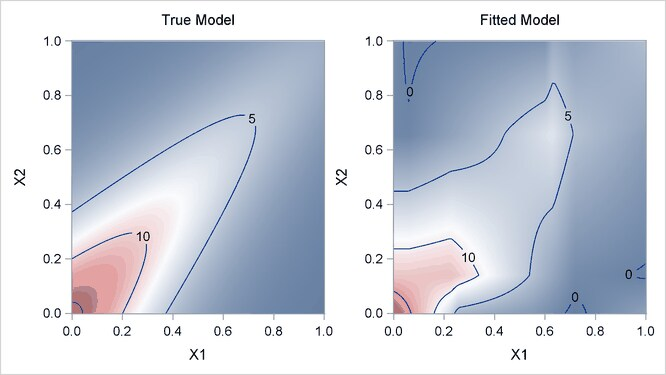For high-dimensional data sets with complex underlying data-generating mechanisms, many different models can almost equally approximate the true mechanisms. Because of the sequential nature of the selection mechanism, any change in intermediate steps due to perturbations from local structures might yield completely different models. Therefore, PROC ADAPTIVEREG might find models that contain noisy variables. For example, if you change the random number seed in generating the data (as in the following statements), PROC ADAPTIVEREG might return different models with more variables. You can use the information from the variable importance table (Output 25.1.5) to aid further analysis.

data artificial;
drop i;
array x{10};
do i=1 to 400;
do j=1 to 10;
x{j} = ranuni(12345);
end;
y = 40*exp(8*((x1-0.5)**2+(x2-0.5)**2))/
(exp(8*((x1-0.2)**2+(x2-0.7)**2))+
exp(8*((x1-0.7)**2+(x2-0.2)**2)))+rannor(1);
output;
end;
run;

proc adaptivereg data=artificial;
model y=x1-x10;
run;


Output 25.1.5 shows that the variables X1 and X2 are two dominating factors for predicting the response, whereas the relative importance of the variable X8 compared to the other two is negligible. You might want to remove the variable if you fit a new model.

Output 25.1.5: Variable Importance